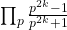# Some remarks concerning the Bernoulli numbers

S. Tabirca
Notes on Number Theory and Discrete Mathematics, ISSN 1310-5132
Volume 6, 2000, Number 2, Pages 29—33

## Details

### Authors and affiliations

S. Tabirca
Department of Computer Science,
Transilvania University, Brasov, Romania

### Abstract

The aim of this article is to propose some remarks on the Bernoulli numbers. Firstly, a simple proof for the the equation B2n+ 1 = 0 is presented. This proof also gives an equation for ζ(2k). Using a simple computation, the values of ζ(2k), k = 1, 12 are presented. Finally, an equation for the infinite productis proposed based on the Bernoulli numbers.

### References

1. T.M.Apostol: A Proof That Euler Missed: Evaluating ζ(2) the Easy Way, Math. Inteligencer 5 (1983), 59-60.
2. M. Bencze: About Bernoulli’s Numbers, Octogon Mathematical Magazine 7 (1999), 151-153.
3. R.Guy: Unsolved Problems in Number Theory, Springer-Verlag, 1994.
4. G.H.Hardy and E.M.Wright: Introduction to the Theory of Numbers, (5th ed.), Oxford University Press, Oxford, 1979.
5. G.A.Jones and J.M.Jones: Elementary Number Theory, Spriger-Verlang, 1998.
6. K. Rosen: Elementary Number Theory and its Application, Addison-Wesley, New York, 1993.
7. I. Tomescu: Introduction to Combinatorics, Wiley, 1987.

## Cite this paper

Tabirca, S. (2000). Some remarks concerning the Bernoulli numbers. Notes on Number Theory and Discrete Mathematics, 6(2), 29-33.